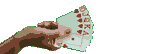# IID Statistics: Independent and Identically Distributed Definition and Examples

Statistics Definitions > IID Statistics

## IID Statistics and Random Sampling

In statistics, we commonly deal with random samples. A random sample can be thought of as a set of objects that are chosen randomly. Or, more formally, it’s “a sequence of independent, identically distributed (IID) random variables”.

In other words, the terms random sample and IID are basically one and the same. In statistics, we usually say “random sample,” but in probability it’s more common to say “IID.”

• Identically Distributed means that there are no overall trends–the distribution doesn’t fluctuate and all items in the sample are taken from the same probability distribution.
• Independent means that the sample items are all independent events. In other words, they aren’t connected to each other in any way.What types of data meet this criteria? Most of the examples you’ll come across in Elementary Statistics are IID. John Mack’s explanation of IID Statistics is clear and easy to grasp:

“A peculiarity of casino games is that they are structured to yield independent, identically-distributed (IID) outcomes. Each iteration of a game–spin of a roulette wheel, roll of dice or deal of shuffled cards–is independent of any other iteration. And the odds of any given result occurring are the same in any iteration. Classical statistics is based on equivalent IID data-generating processes: flipping coins, drawing colored balls from urns, etc.”

## Technically Speaking

A more technical definition of an IID statistics is that random variables X1, X2, . . . , Xn are IID if they share the same probability distribution and are independent events. Sharing the same probability distribution means that if you plotted all of the variables together, they would resemble some kind of distribution: a uniform distribution, a normal distribution or any one of the dozens of other distributions.

Each distribution has its own characteristics. Let’s say we are looking at a sample of n random variables,
X1, X2,…, Xn. Since they are IID, each variable Xi has the same mean (μ), and variance(σ)2. In equation form, that’s:
E(Xi) = μ ; Var(Xi) = σ2
for all i = 1, 2,…, n.
Random variables that are identically distributed don’t necessarily have to have the same probability. A flipped coin can be modeled by a binomial distribution and generally has a 50% chance of a heads (or tails). But let’s say the coin was weighted so that the probability of a heads was 49.5% and tails was 50.5%. Although the coin flips are IID, they do not have equal probabilities.

CITE THIS AS:
Stephanie Glen. "IID Statistics: Independent and Identically Distributed Definition and Examples" From StatisticsHowTo.com: Elementary Statistics for the rest of us! https://www.statisticshowto.com/iid-statistics/
---------------------------------------------------------------------------Need help with a homework or test question? With Chegg Study, you can get step-by-step solutions to your questions from an expert in the field. Your first 30 minutes with a Chegg tutor is free!